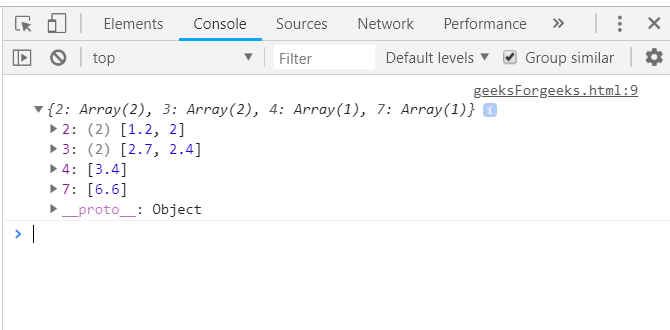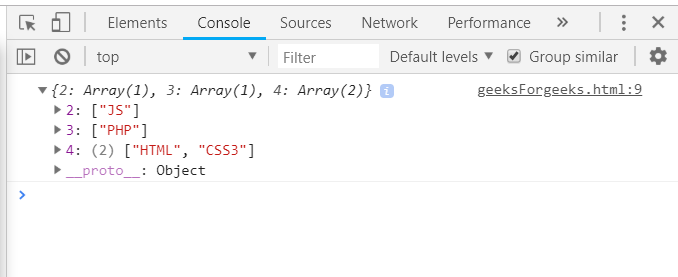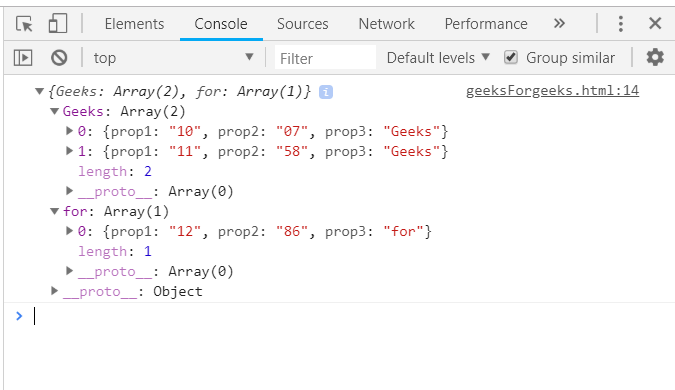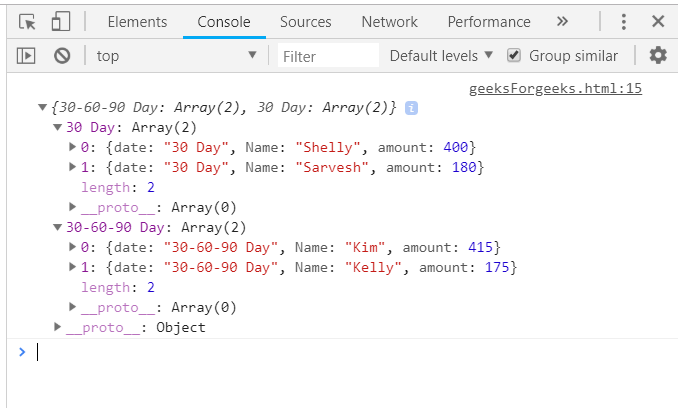# Underscore.js _.groupBy Function

• Last Updated : 24 Nov, 2021

The Underscore.js is a JavaScript library that provides a lot of useful functions that helps in the programming in a big way like the map, filter, invoke etc even without using any built-in objects.
The _.groupBy() function is used to make collection of the elements in the array passed. It works by matching the value of each element to the other. If they matches then they are put in one collection otherwise we will have 2 collections/groups. We can also pass a function based on who’s result we will collect the elements. It can group both on the basis of number and also by string.

Syntax:

`_.groupBy( list, iteratee, context ) `

Parameters: This function accepts three parameters as mentioned above and described below:

• List: This parameter contains the element list.
• Iteratee: This parameter contains the condition which is used to text the elements.
• Context: It is the text which is used to display. It is optional parameter.

Return values: It returns the collections as the different arrays.

Using Math.ceil() in the _.groupBy() function: The _.groupBy() function takes the element from the list one by one and pass it to the Math.ceil() function. Then each elements output of the function will be matched with the output of another element in the Math.ceil() then they are put in 1 group otherwise they will be in 2 separate groups. After all the elements are matched with all the rest elements then the _.groupBy function ends.

Example:

 `<``html``> ` `    ``<``head``> ` `        ``<``script` `type``=``"text/javascript"` `src``= ` `        ``"https://cdnjs.cloudflare.com/ajax/libs/underscore.js/1.9.1/underscore.js"``> ` `        `` ` `    `` ` `     `  `    ``<``body``> ` `        ``<``script` `type``=``"text/javascript"``> ` `            ``console.log(_.groupBy([2.7, 3.4, 6.6, 1.2, 2.0, 2.4], function(num) { ` `                ``return Math.ceil(num);  ` `            ``})); ` `        `` ` `    `` ` `                     `

Output:Using length() in the _.groupBy() function: Passing the array elements to the groupBy() function and match the elements on the basis of their length. If the length of two elements is same then the they will be grouped in 1 group otherwise 2 groups will be formed.

Example:

 `<``html``> ` `    ``<``head``> ` `        ``<``script` `type``=``"text/javascript"` `src``= ` `        ``"https://cdnjs.cloudflare.com/ajax/libs/underscore.js/1.9.1/underscore.js"``> ` `        `` ` `    `` ` `    ``<``body``> ` `        ``<``script` `type``=``"text/javascript"``> ` `           ``console.log(_.groupBy(['HTML', 'CSS3', 'JS', 'PHP'], 'length')); ` `        `` ` `    `` ` ` `

Output:Using a property of the array passed in the _.groupBy() function: First, declare the array (here array is ‘arr’). Choose one condition on which need to check like here ‘prop3’. Then the elements which have the same value in the ‘prop3’ will be grouped in 1 group. Console.log the final answer.

Example:

 `<``html``> ` `    ``<``head``> ` `        ``<``script` `type``=``"text/javascript"` `src``= ` `        ``"https://cdnjs.cloudflare.com/ajax/libs/underscore.js/1.9.1/underscore.js"``> ` `        `` ` `    `` ` `    ``<``body``> ` `        ``<``script` `type``=``"text/javascript"``> ` `            ``var arr =  [ ` `                    ``{prop1:"10", prop2:"07", prop3: "Geeks"}, ` `                    ``{prop1:"12", prop2:"86", prop3: "for"}, ` `                    ``{prop1:"11", prop2:"58", prop3: "Geeks"}  ` `                ``]; ` `               ``console.log(_.groupBy(arr, 'prop3')); ` `        `` ` `    `` ` ` `

Output:Passing ‘date’ as property of the array to the _.groupBy() function together: First define an array with one property as ‘date’ of the format ‘dd-mm-yy’. Then pass the array and the ‘date’ property to the _.groupBy() function. The elements having the same date will be grouped in 1 group. The group numbering will start from 0.

Example:

 `<``html``> ` `    ``<``head``> ` `        ``<``script` `type``=``"text/javascript"` `src``= ` `        ``"https://cdnjs.cloudflare.com/ajax/libs/underscore.js/1.9.1/underscore.js"``> ` `        `` ` `    `` ` `    ``<``body``> ` `        ``<``script` `type``=``"text/javascript"``> ` `               ``var orders = [ ` `                           ``{    date:"30-60-90 Day", Name:"Kim", amount:415     }, ` `                    ``{   date:"30-60-90 Day", Name:"Kelly", amount:175     }, ` `                    ``{   date:"30 Day", Name:"Shelly", amount:400     }, ` `                    ``{   date:"30 Day", Name:"Sarvesh", amount:180     } ` `                    ``]; ` `                ``console.log(_.groupBy(orders, "date")); ` `        `` ` `    `` ` ` `

Output:My Personal Notes arrow_drop_up
Recommended Articles
Page :